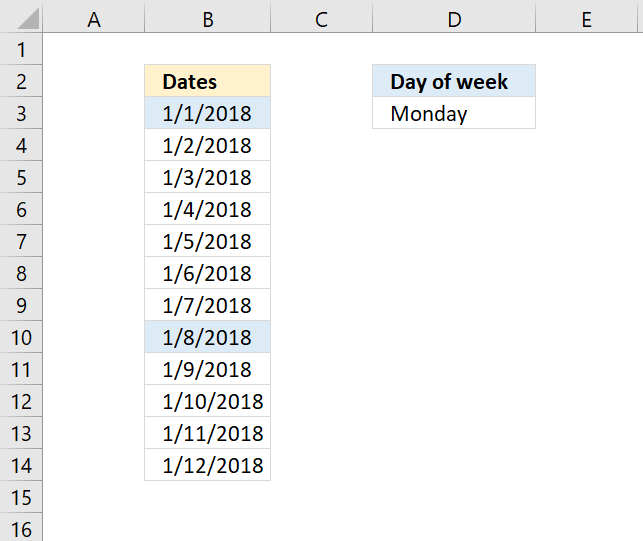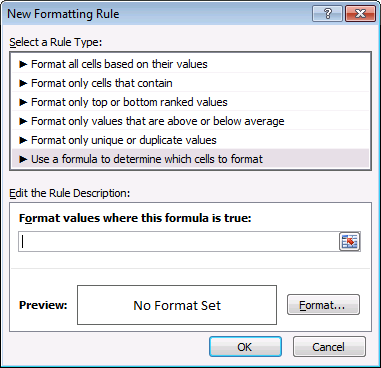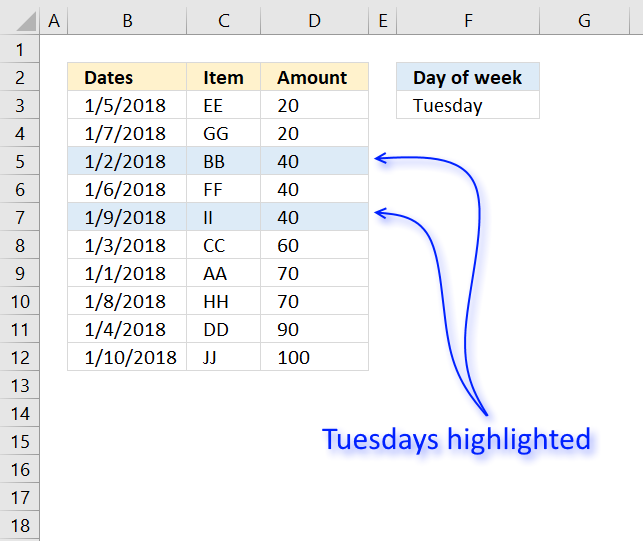Author: Oscar Cronquist Article last updated on November 14, 2018The following conditional formatting formula highlights dates based on day of the week.

=TEXT(B3,"DDDD")=\$D\$3

The TEXT function converts a value to text based on formatting code.  The second argument "DDDD" converts the date to day of week.

TEXT(B3,"DDDD")

becomes

TEXT(43101,"DDDD")

and returns Monday.

The equal sign checks if the value is equal to cell \$D\$3. The dollar signs make sure that cell reference \$D\$3 doesn't change. Each cell in range B3:B14 is evaluated and if they are equal the logical expression returns a boolean value: TRUE or FALSE.

TEXT(B3,"DDDD")=\$D\$3

becomes

"Monday"="Monday" and returns TRUE. This highlights cell B3.

### How to apply conditional formatting to a cell range

1. Select cell range.
2. Go to tab "Home"
3. Click "Conditional Formatting" button.
4. Click "New Rule..."5. Select "Use a formula to determine which cells to format"
6. Type the formula in field "Format values where this formula is true:"
7. Click "Format" button, then pick a formatting.
8. Click OK button.
9. Click OK button.

### Highlight records based on day of weekColumn B contains all the dates we want to be evaluated, we need to make sure that the conditional formatting formula is locked to column B. A dollar sign in front of column B is what we need.

=TEXT(\$B3,"DDDD")=\$D\$3